# TSA Modal Parametric Modeling VI

LabVIEW 2014 Advanced Signal Processing Toolkit Help

Edition Date: June 2014

Part Number: 372656C-01

»View Product InfoDownload Help (Windows Only)

Owning Palette: Modeling and Prediction VIs

Estimates the modal parametric model of a univariate or multivariate (vector) time series. The modal parameters include magnitude, phase, damping factor, and natural frequency. Wire data to the Xt input to determine the polymorphic instance to use or manually select the instance.

Use the pull-down menu to select an instance of this VI.

 Select an instance TSA Modal Parametric Modeling (Waveform)TSA Modal Parametric Modeling (Array)TSA Vector Modal Parametric Modeling (Waveform)TSA Vector Modal Parametric Modeling (Array)

## TSA Modal Parametric Modeling (Waveform)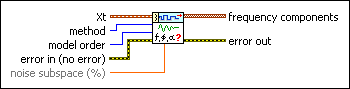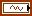Xt specifies the univariate time series.method specifies the method to use in estimating the frequency component of the time series.

 0 Prony (default)—Computes parameters of a modal parametric model by solving complex exponential equations in the lease-square sense. This method also is called the Least-Square Complex Exponential method. 1 Matrix Pencil—Computes parameters of a modal parametric model by solving complex exponential equations in the lease-square sense. This method is a modified Prony method with noise disturbances considered.model order specifies the model order. The value of model order must be at least twice the number of frequency components you want to estimate. The default is 4.error in describes error conditions that occur before this node runs. This input provides standard error in functionality.noise subspace specifies the percentage of frequency components due to noise in the time series. The default is 50. This option is available only when method is Matrix Pencil.frequency components returns information about the estimated frequency components.frequency returns the estimated frequency of the frequency component.damping factor returns the estimated damping factor of the frequency component.magnitude returns the estimated magnitude of the frequency component.phase returns the estimated phase of the frequency component.error out contains error information. This output provides standard error out functionality.

## TSA Modal Parametric Modeling (Array)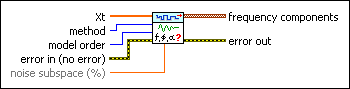Xt specifies the univariate time series.method specifies the method to use in estimating the frequency component of the time series.

 0 Prony (default)—Computes parameters of a modal parametric model by solving complex exponential equations in the lease-square sense. This method also is called the Least-Square Complex Exponential method. 1 Matrix Pencil—Computes parameters of a modal parametric model by solving complex exponential equations in the lease-square sense. This method is a modified Prony method with noise disturbances considered.model order specifies the model order. The value of model order must be at least twice the number of frequency components you want to estimate. The default is 4.error in describes error conditions that occur before this node runs. This input provides standard error in functionality.noise subspace specifies the percentage of frequency components due to noise in the time series. The default is 50. This option is available only when method is Matrix Pencil.frequency components returns information about the estimated frequency components.frequency returns the estimated frequency of the frequency component.damping factor returns the estimated damping factor of the frequency component.magnitude returns the estimated magnitude of the frequency component.phase returns the estimated phase of the frequency component.error out contains error information. This output provides standard error out functionality.

## TSA Vector Modal Parametric Modeling (Waveform)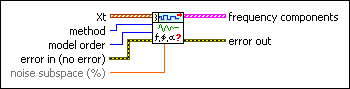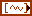Xt specifies the multivariate (vector) time series.method specifies the method to use in estimating the frequency component of the time series.

 0 Prony (default)—Computes parameters of a modal parametric model by solving complex exponential equations in the lease-square sense. This method also is called the Least-Square Complex Exponential method. 1 Matrix Pencil—Computes parameters of a modal parametric model by solving complex exponential equations in the lease-square sense. This method is a modified Prony method with noise disturbances considered.model order specifies the model order. The value of model order must be at least twice the number of frequency components you want to estimate. The default is 4.error in describes error conditions that occur before this node runs. This input provides standard error in functionality.noise subspace specifies the percentage of frequency components due to noise in the time series. The default is 50. This option is available only when method is Matrix Pencil.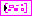frequency components returns information about the estimated frequency components.frequency returns the estimated frequency of the frequency component.damping factor returns the estimated damping factor of the frequency component.magnitude returns the estimated magnitude of the frequency component. Each element of the array represents one channel series in Xt.phase returns the estimated phase of the frequency component. Each element of the array represents one channel series in Xt.error out contains error information. This output provides standard error out functionality.

## TSA Vector Modal Parametric Modeling (Array)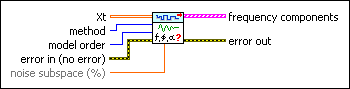Xt specifies the multivariate (vector) time series. Each column of the 2D array represents a vector at certain time.method specifies the method to use in estimating the frequency component of the time series.

 0 Prony (default)—Computes parameters of a modal parametric model by solving complex exponential equations in the lease-square sense. This method also is called the Least-Square Complex Exponential method. 1 Matrix Pencil—Computes parameters of a modal parametric model by solving complex exponential equations in the lease-square sense. This method is a modified Prony method with noise disturbances considered.model order specifies the model order. The value of model order must be at least twice the number of frequency components you want to estimate. The default is 4.error in describes error conditions that occur before this node runs. This input provides standard error in functionality.noise subspace specifies the percentage of frequency components due to noise in the time series. The default is 50. This option is available only when method is Matrix Pencil.frequency components returns information about the estimated frequency components.frequency returns the estimated frequency of the frequency component.damping factor returns the estimated damping factor of the frequency component.magnitude returns the estimated magnitude of the frequency component. Each element of the array represents one channel series in Xt.phase returns the estimated phase of the frequency component. Each element of the array represents one channel series in Xt.error out contains error information. This output provides standard error out functionality.

## TSA Modal Parametric Modeling Details

For a univariate impulse response time series, this VI estimates the modal parametric model according to the following equation: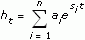where ht is the univariate impulse response series, and n is the model order.

ai denotes one of the complex amplitudes, which is defined as:

ai = riejq

where r is magnitude, and q is phase.

Si is one of the modal poles, which is defined as:

Si = a + j2pf

where a is damping factor, and f is frequency.

For a multivariate impulse response time series, this VI estimates the modal parametric model according to the following equation: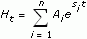where Ht is the multivariate impulse response series. Ht is a k×1 vector with k variables that come from k sources. Ai is a k×1 complex amplitude vector with k variables. AiT=(a1i,…,aki). Si is one of the modal poles. n is the model order.

Refer to the univariate modal parametric model for the descriptions of aki in the vector Ai.

### Reference:

Hua, Y., and T. K. Sarkar. 1990. "Matrix Pencil Method for Estimating Parameters of Exponentially Damped/Undamped Sinusoids in Noise." IEEE Transaction on Acoustic, Speech, and Signal Processing vol. 38.5: 814-824.

## Examples

Refer to the following VIs for examples of using the TSA Modal Parametric Modeling VI:

• Modal Analysis of a Plate VI: labview\examples\Time Series Analysis\TSAApplications
• Frequency Components VI: labview\examples\Time Series Analysis\TSAGettingStarted